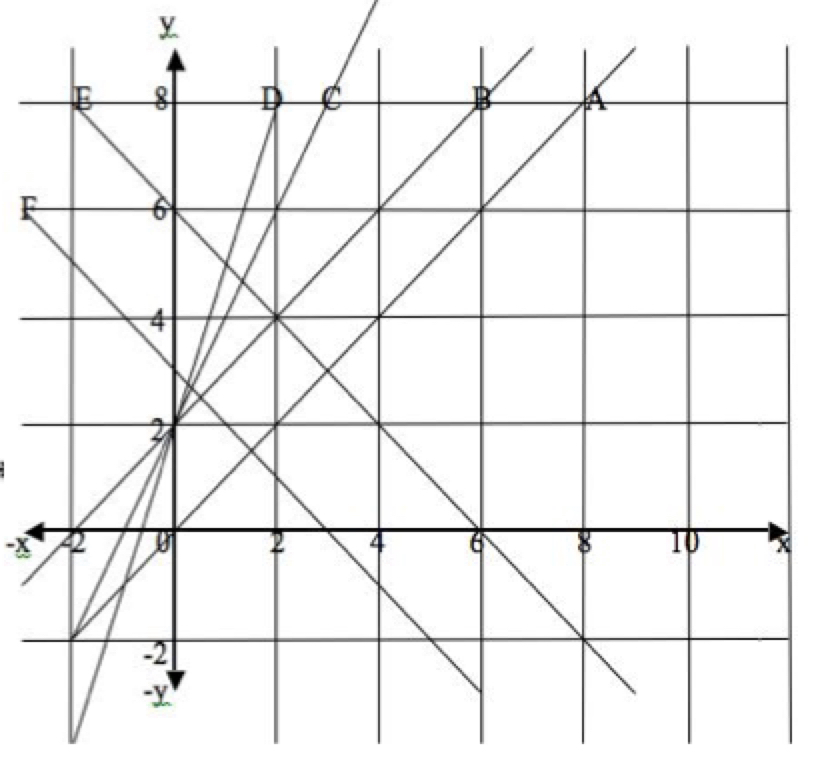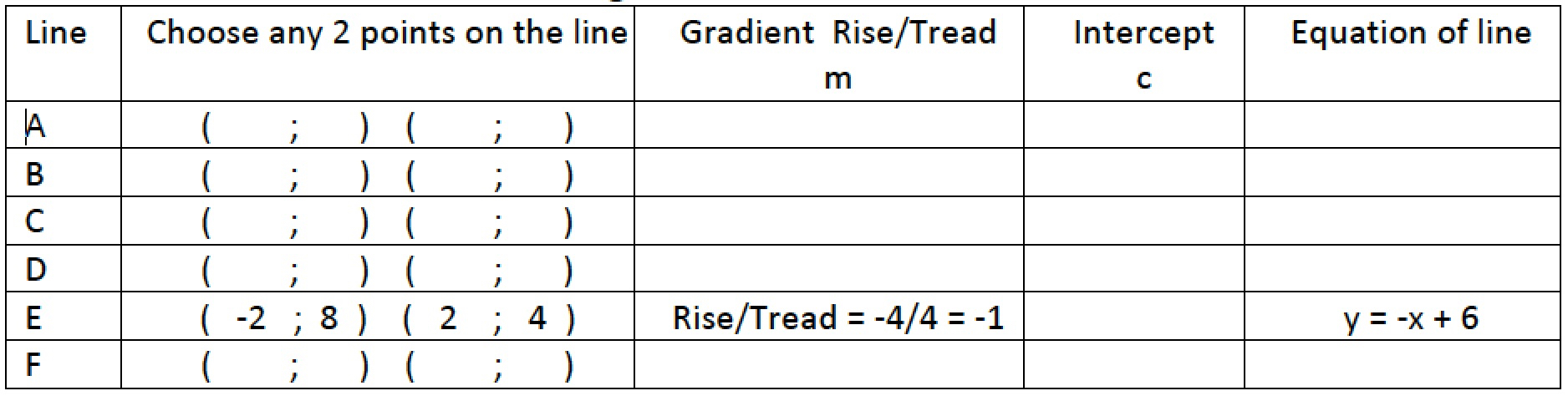1. For each line fill in your answers to the following questions in the table below: (Line E has been done for you.)

(i) Write down the coordinates (0; c) of the point of intersection of the line with the y-axis (the y intercept).

(ii) Choose two points on the line $(x_1;y_1), (x_2;y_2)$. Find the gradient of the line from the formula $m=\frac{y_2-y_1}{x_2-x_1}$.

(iii) Use the values of c and m you have found to write the equation of the line in the form $y = mx + c$.

2. What do you notice when you compare the equations of these lines?3. Copy the graphs below and fill in the coordinates of the points.

What are the gradients of the x-axis, the y-axis and the other 4 lines shown?

Write down the equations of the x-axis, the y-axis and the other 4 lines shown.

See the Notes for Teachers

Tagged with:

### One Response to Lines

1.Nontsikelelo says:

Thanks a lot for seeing this site. I will visit it frequently.